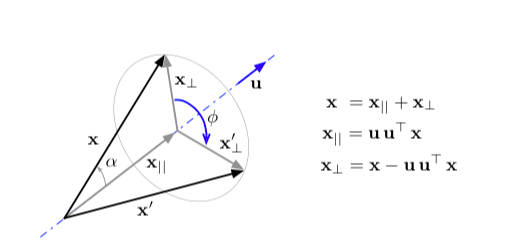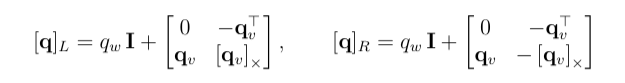## 平面旋转

\begin{aligned} 1 \rightarrow \cos \theta + i \sin \theta \\ i \rightarrow - \sin \theta + i \cos \theta \end{aligned}

\begin{aligned} &x(\cos \theta + i \sin \theta) + y (-\sin \theta + i \cos \theta)\\ =& x(\cos \theta + i \sin \theta) + i y ( i\sin \theta + \cos \theta) \\ =& (x + iy) (\cos \theta + i \sin \theta) \\ =& (x + iy) e^{i\theta} \end{aligned}

## 3D旋转

$e^{\mathbf{u}\theta} = \cos \theta + \mathbf{u} \sin \theta$$1 \rightarrow \cos \theta + i \sin \theta$

$\mathbf{x}_{\perp} \rightarrow \mathbf{x}_{\perp} \cos \phi + (\mathbf{u} \times \mathbf{x}_{\perp}) \sin \phi$

$\mathbf{x}' = \mathbf{x}_{\parallel} + \mathbf{x}_{\perp} \cos \phi + (\mathbf{u} \times \mathbf{x}_{\perp}) \sin \phi$$[e^{\mathbf{u}\theta}]_L [e^{-\mathbf{u}\theta}]_R = \begin{bmatrix} 1 & 0 \\ 0 & \cos^2 \theta + \sin2\theta [\mathbf{u}]_{\times} + (\mathbf{uu}^T + [\mathbf{u}]_{\times}[\mathbf{u}]_{\times})\sin^2\theta \end{bmatrix}$

\begin{aligned} &\mathbf{x} \cos^2 \theta + \sin2\theta [\mathbf{u}]_{\times} \mathbf{x} + (\mathbf{uu}^T \mathbf{x} + [\mathbf{u}]_{\times}[\mathbf{u}]_{\times}\mathbf{x})\sin^2\theta \\ = &\mathbf{x} \cos^2 \theta + (\mathbf{u} \times \mathbf{x}_{\perp}) \sin2\theta + (\mathbf{uu}^T \mathbf{x}_{\parallel} + [\mathbf{u}]_{\times}[\mathbf{u}]_{\times}\mathbf{x}_{\perp})\sin^2\theta \\ = & \mathbf{x} \cos^2 \theta + (\mathbf{u} \times \mathbf{x}_{\perp}) \sin2\theta + \mathbf{x}_{\parallel} \sin^2\theta - \mathbf{x}_{\perp} \sin^2\theta \\ = & \mathbf{x}_{\parallel} + \mathbf{x}_{\perp}\cos2\theta + (\mathbf{u} \times \mathbf{x}_{\perp}) \sin2\theta \end{aligned}

$e^{\mathbf{u}\theta/2} \otimes \mathbf{x} \otimes e^{-\mathbf{u}\theta/2}$

NOTE：很遗憾，目前为止也只是抽象推导了四元数旋转，还是没有对四元数旋转得到更加直观的理解。3D旋转与虚数的关系并没有2D来的那么直观。相关理解可能后续有时间再继续进行吧。

## 参考文献

B. V. Adorno, “Robot Kinematic Modeling and Control Based on Dual Quaternion Algebra—Part I: Fundamentals.,” 2017.

J. Sola, “Quaternion kinematics for the error-state KF,” p. 73.